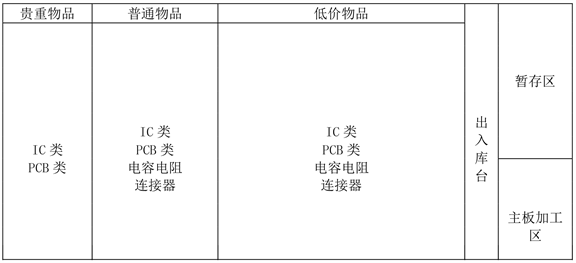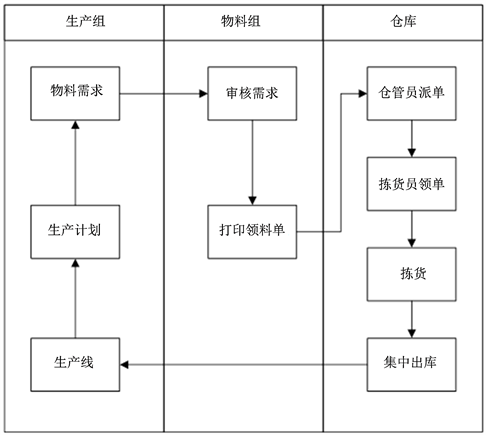# A公司零部件仓库货位优化研究Optimization Research on Parts Warehouse Slotting of Company A

• 全文下载: PDF(568KB)    PP.28-37   DOI: 10.12677/MSE.2019.81005
• 下载量: 348  浏览量: 1,520

This paper takes the server parts of company A as the research object, and studies the optimization of the location allocation of the server parts warehouse. It analyzed the server component position distribution optimization principle. Combining with the actual situation to establish A company goods allocation optimization mathematical model, the operator of the genetic algorithm is designed. By using the software of Matlab implementation model, it is concluded that the feasible optimization scheme would be to verify the effectiveness of the designed by genetic algorithm, and has reference significance to the similar problem.

1. 引言

Van  在自动拣货系统的货位优化研究中，基于分类存储策略，以拣货时间最短为目标构建了单目标货位优化模型，并运用遗传算法进行求解，通过对拣货时间进行效果分析，验证了该方法有效的减少了拣货时间。罗键等  为了提高自动化立体仓库小车存取效率，以自动拣货小车拣货时间最短为目标函数，建立了单目标系统货位优化的数学模型，并基于离散粒子群算法实现了货位分配的优化，有效地缩短小车拣货时间。李珍萍  以人工拣选仓库或配送中心某一段时期内的历史订单为研究对象，建立了以人工订单拣选作业导致的人体能耗最小为目标的单目标货位优化模型，并利用贪婪算法对其进行快速求解，并验证了该方法能有效降低人工劳动强度，提高劳动效率。

Muppani  将货物的存储空间与货物周转率的比值放入货位分配模型中，以存储和拣选成本最低建立模型，并运用遗传算法进行求解，并验证了优化后的货位分配结果比原货位分配方式更优。Mantel  提出面向订单的货位指派方法，对一定周期的订单进行分析，在穿越拣选策略的条件下，考虑货品周转率和相关性，以拣货时间最短和同类物品距离最近为目标，将该问题抽象成线性整数规划模型，并用遗传算法求解上述模型。李霞  以自动化立体仓库为研究对象，对其货品的货位分配进行优化研究，考虑货架稳定性和出库频率，建立从入库、出库到倒库三个阶段的多目标动态货位优化模型，采用基于权重系数变换法的多目标遗传算法求解模型，并对仿真结果进行分析讨论，验证了算法的有效性。张子才  研究自动化仓库货位优化问题，提出了一种新的货位优化方法，综合考虑货物周转率及相关性因素，建立了多目标货位优化问题解析模型，并lingo优化工具进行了仿真分析。仿真分析结果表明，所提出的货位优化方案极大地提高了仓库的存储效率及存储利用率。郭有俊  根据电子元器件分销商区域配送中心的特点，运用聚类算法对元器件进行分析，并在此基础上构建了货位优化数学模型，运用贪婪算法进行求解，用实例证明优化后的储位分配方法可以以缩短拣货人员在货物拣取时的行走距离和作业时间，提高配送中心的作业效率。祖巧红  以立体仓库库存为研究对象，研究了货位分配优化问题。分析了汽车零部件货位布局优化原则，建立多目标货位分配优化数学模型，运用Matlab软件实现模型的求解，得出可行的货位优化方案。最后结合实例进行多目标货位优化数学模型求解及应用，并以三维仿真图形展示了优化效果，验证了所设计的遗传算法的有效性。夏云飞  以S企业零部件存储区为主要研究对象，对该公司的货分配问题，出货量以及出货频次为依据，运用EIQ分析法对零部件进行了ABC分类，优先将A类货品存放在离出库口较近的位置，B类其次，C类最后，从而使得整体出库时间达到最短。并以拣货时间最少，相关性大零部件距离最短、优化前后货物移动距离最小建立了多目标货位优化模型。并通过设计遗传算法多模型进行求解，对比了迭代前后货物距出入口的距离，证明了该方法的有效性。

2. 企业背景

A公司是一家大型生产企业，主要从事服务器及网络设备的生产制造，A公司零部件仓库占地约2000平方米，存储的零部件主要有IC类、PCB类、电容、电阻、连接器五大类，零部件种类繁多，数量庞大，体积差异明显。目前，A公司零部件仓库采用的是定位存储的存储策略，将五个大类零部件按照金额分为贵重物品、普通物品和低价物品三类，并将其分别存放在三个区域。仓库零部件以整箱的方式存放在4层搁板式货架上，经实地调查了解到仓库每个货架的长、宽、高分别为4 m × 1 m × 2 m，每个货架共有四层五列，共有货位26,000个，每个货位按照字母和数字的组合来编码。A公司零部件仓库布局见图1Figure 1. A company parts warehouse layout mapFigure 2. A company parts warehouse picking operation process

3. 多目标货位优化模型的构建

3.1. 模型假设及符号说明

3.1.1. 模型假设

1) 货位的数量都是固定的，不能超过原有的排、列、层数，当货物入库的时候，必须是在有货位的情况下，并且入库的货物应小于等于货位数。

2) 决策变量的限制，即货位坐标必须为整数。

3.1.2. 符号说明

1) ${D}_{i}$ ——零部件i到出入口的距离；

2) $n$ ——共有n种零部件；

3) ${p}_{i}$ ——货物i的周转率；

4) ${M}_{i}$ ——零部件i的质量；

5) $\text{u}$ ——设备与仓库地面的摩擦系数；

6) $\text{g}$ ——重力加速度；

7) ${y}_{i}$ ——零部件i所在的层数；

3.2. 模型构建

3.2.1. 目标函数的构建

1) 要提高仓库拣货作业效率，应减短货物拣选时工作人员的行走距离。假设仓库有n件货物需要进行拣选操作，其中货物i的移动距离为横向、纵向和垂直距离之和那么最小化距离表达如下：

$\text{D}\left(\text{r},\text{s},\text{t}\right)=\mathrm{min}{\sum }_{i=1}^{n}{r}_{i}\ast L+{s}_{i}\ast H+{t}_{i}\ast W$ (1)

${f}_{1}\left(r,s,t\right)=\mathrm{min}{\sum }_{i=1}^{n}\left({r}_{i}\ast L/{v}_{x}+{s}_{i}\ast H/{v}_{y}+{t}_{i}\ast W\right)/{v}_{z}$ (2)

2) 为了提高空间利用率，对周转率高的货物，应该放置在离出入库较近的地方，综合以上两点，拣选时间最优的表达式如下：

${f}_{2}\left(r,s,t\right)=\mathrm{min}{\sum }_{i=1}^{n}{p}_{i}\left({r}_{i}\ast L/{v}_{x}+{s}_{i}\ast H/{v}_{y}+{t}_{i}\ast W/{v}_{z}\right)$ (3)

3) 为了保证货架承载受力均勾，所有的货物进行放置的时候都需要考虑货位的承受能力，将重量大的货物放在下面，货物轻的放置在上面的原则来存放货物。货架的稳定性与重心密切相关，货架的重心越小，稳定性越高，所以构造目标函数，使得重心最小，表达如下：

${f}_{3}\left(r,s,t\right)=\mathrm{min}{\sum }_{i=1}^{n}{p}_{i}\left({M}_{i}\ast {y}_{i}\ast H\right)/{\sum }_{i=1}^{n}{M}_{i}$ (4)

4) 拣选作业的效率，还和人体的耗能息息相关，A公司仓库零部件的拣选作业中，基本都靠人工作业，所以人体的耗能最小也可以提高作业效率到达优化的目标。以人工拣选领料单作业导致人体耗能最小为目标，表达式如下：

${f}_{4}=\mathrm{min}{\sum }_{i=1}^{n}u{M}_{i}\ast g\left({r}_{i}\ast L+{s}_{i}\ast H+{t}_{i}\ast W\right)$ (5)

3.2.2. 约束条件

1) $1\le \text{r}\le \text{x}$

2) $1\le \text{s}\le \text{y}$

3) $1\le \text{t}\le \text{z}$

4. 模型求解

4.1. 确定目标函数权重

$\text{f}=\mathrm{min}\left(a{f}_{1}+b{f}_{2}+c{f}_{3}+d{f}_{4}\right)$ (6)Table 2. Indicator value of each evaluation factor

$\left[\begin{array}{cccc}1& 3& 5& 5\\ 1/3& 1& 3& 5\\ 1/5& 1/3& 1& 1\\ 1/5& 1/5& 1/3& 1\end{array}\right]$

4.2. 遗传算法设计

1) 编码

1) 一条染色体代表一种货位的分配方案；

2) 每条染色体中的基因数目就代表着货位优化区域内货物的个数；

3) 基因的值就代表货物存储的货位。

2) 确定使用度函数

$\text{F}=1/a\left({f}_{1}+1\right)+b\left({f}_{2}+1\right)+c\left({f}_{3}+1\right)+d\left({f}_{4}+1\right)$ (7)

3) 种群的初始化

4) 遗传优化算法的主要操作算子

① 选择操作

② 交叉操作

③ 变异操作

4.3. 仿真实验Table 3. Simulation basic parameters

5. 结论

  Van Den Berg, J.P. and Gademann, A. (2000) Simulation Study of an Automated Storage/Retrieval System. International Journal of Production Research, 38, 1339-1356. https://doi.org/10.1080/002075400188889  罗键, 钟寿桂, 吴长庆. 基于离散粒子群算法的AVS/RS货位优化[J]. 厦门大学学报(自然科学版), 2009, 48(2): 212-215.  李珍萍, 于洋涛, 李文玉. 基于贪婪算法的仓库货位优化问题研究[J]. 物流技术, 2015, 34(5): 242-244.  Muppani (Muppant), V.R. and Adil, G.K. (2008) A Branch and Bound Algorithm for Class Based Storage Location Assignment. European Journal of Operational Research, 189, 492-495. https://doi.org/10.1016/j.ejor.2007.05.050  Mantel, C.J. (1996) An Integrated Storage System Evaluation Model. Applied Mathematical Modelling, 20, 359-370. https://doi.org/10.1016/0307-904X(95)00128-7  李霞, 朱煜明. 自动化立体仓库动态货位分配建模与仿真[J]. 物流科技, 2016, 39(3): 131-136, 151.  张子才, 李奎. 基于lingo算法的货位优化研究[J]. 宝钢技术, 2015(1): 1-4.  郭有俊. 某电子元器件分销配送中心的货位优化研究[J]. 企业技术开发, 2013, 32(9): 6-9, 47.  祖巧红, 徐晓霞, 毛一超. 汽车零部件立体仓库货位优化问题研究[J]. 图学学报, 2015, 36(2): 312-317.  夏云飞. 基于多目标模型的S企业零部件存储区货位优化研究[D]: [硕士学位论文]. 北京: 北京交通大学, 2017.